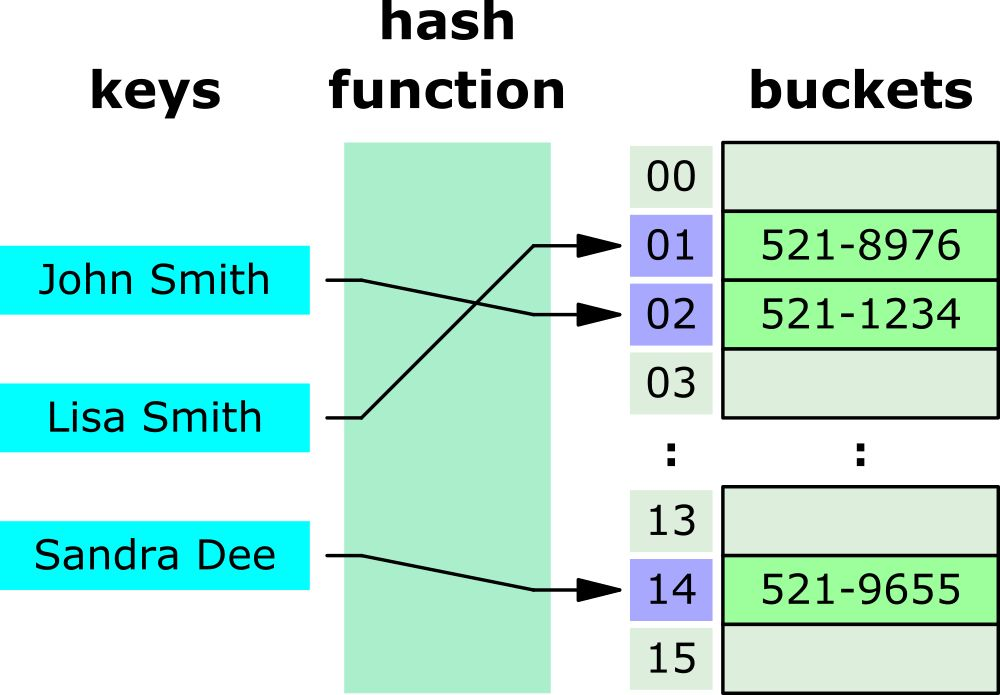×

Search anything:

# Built-in Hash Table in JavaScript

#### JavaScript Data StructuresGet this book -> Problems on Array: For Interviews and Competitive Programming

Hash tables are a popular data structure used in computer programming to store and retrieve data quickly. A hash table uses a hashing function to map keys to values, which can be accessed in constant time. In this article, we will explore how hash tables work in JavaScript, with examples and tips.

Built-in Hash Table in JavaScript involve using a Map with 2 key methods set and get.

## What is a hash table?

A hash table is a data structure that uses a hashing function to map keys to values. The hashing function takes a key as input and returns an index in the table where the value is stored. The index is calculated based on the key's hash code, which is a numeric representation of the key.

The hash code should be deterministic, which means that the same key should always produce the same hash code. This is important because if two different keys produce the same hash code, it is called a collision, and the hash table needs to resolve the collision to store both values correctly.

Hash Table Time Complexity In Big O Notation

Algorithm Average Worst case
Delete O(1) O(n)
Space O(n) O(n)
Search O(1) O(n)
Insert O(1) O(n)

A Hash Table uses a hash function to convert a key into an integer index, which is then used to determine where to store the associated key/value pair in memory.## How to implement a hash table in JavaScript

In JavaScript, a hash table can be implemented using an object or a Map. An object is a built-in data type in JavaScript, and it can be used to store key-value pairs. A Map is a data structure introduced in ES6, and it provides more functionality than an object, such as the ability to store any data type as a key.

Here is an example of how to create a hash table using an object:

``````const hashTable = {};
hashTable['name'] = 'John';
hashTable['age'] = 30;
hashTable['gender'] = 'male';

console.log(hashTable['name']); // Output: John
console.log(hashTable['age']); // Output: 30
console.log(hashTable['gender']); // Output: male
``````

In this example, we create a hash table using an object, and we add three key-value pairs to the table using bracket notation. We can retrieve the values from the table using bracket notation as well.

Here is an example of how to create a hash table using a Map:

``````const hashTable = new Map();

hashTable.set('name', 'John');
hashTable.set('age', 30);
hashTable.set('gender', 'male');

console.log(hashTable.get('name')); // Output: John
console.log(hashTable.get('age')); // Output: 30
console.log(hashTable.get('gender')); // Output: male
``````

In this example, we create a hash table using a Map, and we add three key-value pairs to the table using the set() method. We can retrieve the values from the table using the get() method.

## Tips for using hash tables in JavaScript

Here are some tips for using hash tables in JavaScript:

1. Choose a good hashing function: A good hashing function should produce a unique hash code for each key to minimize collisions. There are many hashing functions to choose from, and some of them are built into JavaScript, such as the hash() method.
2. Be aware of collisions: Collisions can occur when two different keys produce the same hash code. When a collision occurs, the hash table needs to resolve the collision to store both values correctly. One common way to resolve collisions is to use separate chaining, where each table index contains a linked list of values.
3. Use Map for more functionality: If you need more functionality than an object provides, such as the ability to store any data type as a key, use a Map instead.
4. Use hash tables for fast lookups: Hash tables are designed for fast lookups, so use them when you need to retrieve values quickly based on a key.

## Conclusion

Hash tables are a powerful data structure for storing and retrieving data quickly. In JavaScript, hash tables can be implemented using an object or a Map. When using hash tables, be sure to choose a good hash function that distributes the keys evenly across the hash table to avoid collisions and maintain efficiency.#### Sentayhu Berhanu

Sentayhu Berhanu is a Full-stack web developer, dedicated and efficient in software development with over 2 years of experience and has completed my BSc degree in Computer Science.